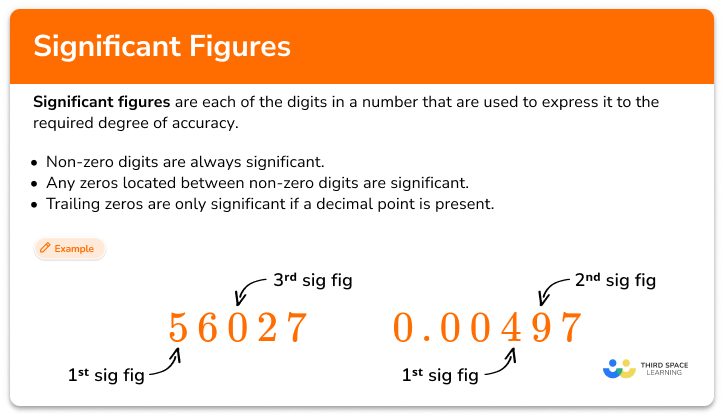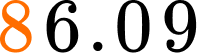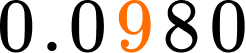# Significant figures

Here you will learn about significant figures, how to identify significant figures within a given number and how to round to one significant figure, two significant figures, and three significant figures.

Students will first learn about significant figures as part of expression and equations in the 8th grade.

## What are significant figures?

Significant figures are each of the digits in a number that are used to express it, to the required degree of accuracy, starting from the first non-zero digit. The first significant figure, or significant digit, of a number is the most important digit because it expresses the size of the number.

Significant figures are often used with measured values.

To locate a significant figure, you need to find the first non-zero digit. This is the first significant figure. The next digit is the second significant figure and so on.

A significant figure could be to the left of the decimal point or the right of the decimal point.

There are significant figure rules that will help you identify all the significant figures in a given number:

• Non-zero digits are always significant.
• Any zeros located between non-zero digits are significant.
• Trailing zeros are only significant if a decimal point is present.

For example,

Rounding numbers to significant figures (often abbreviated to sig figs or s.f.), is similar to rounding to a number of decimal places, such as the ones place, tens place, hundreds place, and thousands place.

### What are significant figures?## Common Core State Standards

How does this relate to 8th grade math?

• Grade 8: Expressions and Equations (8.EE.A.4)
Perform operations with numbers expressed in scientific notation, including problems where both decimal and scientific notation are used. Use scientific notation and choose units of appropriate size for measurements of very large or very small quantities (for example, use millimeters per year for seafloor spreading). Interpret scientific notation that has been generated by technology.

## How to identify significant figures

In order to identify significant figures:

1. Find the first non-zero digit.
2. Starting at the first non-zero digit, count the number of digits that are non-zero numbers or zeros between non-zero digits.
3. If there is a decimal present, count trailing zeros.
4. Label the number of significant figures.

## Significant figures examples

### Example 1: significant figures

Identify the correct number of significant figures in the number 405.

1. Find the first non-zero digit.

The first non-zero digit is the 4 in the hundreds place.

2Starting at the first non-zero digit, count the number of digits that are non-zero numbers or zeros between non-zero digits.

All three digits are either non-zero numbers or have a zero between non-zero digits.

The 4 is the first significant digit, and the 5 is the last significant digit.

3If there is a decimal present, count trailing zeros.

There are no decimal points or trailing zeros present.

4Label the number of significant figures.

The number 405 has 3 significant figures.

### Example 2: significant figures

Identify the correct number of significant figures in the number 0.037.

The first non-zero digit is the 3 in the hundredths place.

The 3 and the 7 are non-zero digits. The 3 is the first significant digit, and the 7 is the last significant digit.

There is a decimal in this number, but no trailing zeros.

The number 0.037 has two significant figures.

### Example 3: significant figures

Calculate the number of significant figures for the number 0.004500.

The first non-zero digit is the 4 in the thousandths place.

The 4 is the first significant digit, and the 5 is the last significant non-zero digit.

There is a decimal present, so the last 2 zeros in the number are trailing zeros.

The number 0.004500 has four significant figures.

## How to round to significant figures

In order to round to a given number of significant digits:

1. Locate the significant figure for the degree of accuracy required.
2. Look at the next digit immediately to the right. When the digit is \bf{5} or more, round up by adding \bf{1} to the previous digit. When the digit is less than \bf{5} , round down by keeping the previous digit the same.
3. When the degree of accuracy is \bf{10} or more, use zeros as place holders.

### Example 4: rounding to one significant figure

Round 3,692 to one significant figure (1 \, s.f) .

The first non-zero digit is 3, therefore 3 is the first significant figure.

6 is more than 5.

Because 6 is greater than 5, you will round up. Adding 1 to the 3 gives you 4.

The first significant figure represents thousands, so you must include three zeros as place holders to make it the correct size.

3,692 is \bf{4,000} to 1 \, s.f.

### Example 5: rounding to two significant figures

Round 0.07039 to two significant figures (2 \, s.f.) .

The first non-zero digit is the 7, which is the first significant figure; therefore, the 0, at the thousandths place, is the second significant figure.

3 is immediately to the right.

3 is less than 5.

Because 3 is less than 5, you will round down.

0.07039 is \bf{0.070} to 2 \, s.f.

It is important to keep the zero after the seven as it must be given to two significant figures.

Note: You will often write very small numbers, such as 0.07039 using standard form (scientific notation).

0.07039 can be written as 7.039 \times 10^{-2}

### Example 6: rounding to three significant figures

Round 24.753 to three significant figures (3 \, s.f.) .

The first non-zero digit is 2, which is the first significant figure. Therefore, the 7, in the tenths place, is the third significant figure.

The digit to the right is 5.

Because the number is 5, you will round up. Adding 1 to the 7 gives you 8.

You do not need to include any zero place holders after the 8 because there are already three significant figures present.

24.7 is \bf{24.8} to 3 \, s.f.

### Teaching tips for significant figures

• When rounding to a given significant figure, students may struggle with numbers with more digits. Having students underline or color code the significant figure they are rounding to will help them visually see the place, and not get lost in all of the digits.

• Rounding errors are common when rounding to significant figures. For some students, posting of common rounding rules may help keep this from happening.

### Easy mistakes to make

• Assuming the first zeros are significant
A common error is to think the first zeros in a number like 0.00467 are significant. These are called leading zeros and do not tell you the size of the number. The first non-zero digit is 4, and tells you that the number is roughly 4 thousandths.

• Assuming all zeros are not significant
A common error is to think that all the zeros in a number like 9,006 are not significant. Once the first significant figure has been located, all digits after are significant. Otherwise, you might round to the incorrect number of significant figures.

• Leaving out zeros to make the number the correct size
It is important to remember to fill in the zeros if the degree of accuracy is greater than 10. A common mistake when rounding a number like 378 to two significant figures is to say it is 38 and not 380.

This significant figures topic guide is part of our series on rounding decimals. You may find it helpful to start with the main rounding decimals topic guide for a summary of what to expect or use the step-by-step guides below for further detail on individual topics. Other topic guides in this series include:

### Practice significant figures questions

1. Identify the correct number of significant figures in the number 86.09.

1 significant figure3 significant figures4 significant figures2 significant figuresThe first non-zero number is 8.Continue counting all non-zero digits and zeros between non-zero digits.

All 4 digits are significant figures.

The number 86.091 has 4 significant figures.

2. Identify the correct number of significant figures in the number 0.0980.

2 significant figures3 significant figures4 significant figures5 significant figuresThe first non-zero number is 9.Continue counting all non-zero digits and zeros between non-zero digits.

The zero in the ten thousandths place will also be a significant figure because it’s a trailing zero when there is a decimal present.

The number 0.0980 has 3 significant figures.

3. Which of the following numbers has the fewest significant figures?

2.340.50121.40900.00000564. Round 76,340 to one significant figure.

870,00080,0008,000The first significant figure is the 7.The next digit to the right is 6, which is bigger than 5, so you round up.

Adding 1 to 7 gives you 8 and filling in the placeholder zeros gives you 80,000.

Rounding off 76,340 to one significant figure is 80,000.

5. Round 78.56 to two significant figures.

78.6787980The first significant figure is 7 and the second is 8.The next number to the right is 5, so you round up.

Adding one to the 8 is 9, which gives you 79.

Rounding off 78.56 to two significant figures is 79.

6. Round 0.0051489 to three significant figures.

0.0050.005150.005140.00525The first significant figure is 5 and the third significant figure is 4.The next number to the right is 8, which is bigger than 5, so you round up.

Adding 1 to the 4 is 5, which gives you 0.00515.

Rounding off 0.0051489 to three significant figures is 0.00515.

## Significant figures FAQs

How do I calculate significant figures?

All non-zero digits are significant figures. Zeros are also significant with the exception of two things:
1) the zero is alone before the decimal point and,
2) the zero is after the decimal point, but before the first non-zero digit.

How do I round to a significant figure?

You will first identify the significant figure that you will round to.
Follow the same rounding steps as you have learned with whole numbers and decimal places. (For example, 5 or more, you add one to the digit of the rounded place. 4 or less, the digit will stay the same).

## Still stuck?

At Third Space Learning, we specialize in helping teachers and school leaders to provide personalized math support for more of their students through high-quality, online one-on-one math tutoring delivered by subject experts.

Each week, our tutors support thousands of students who are at risk of not meeting their grade-level expectations, and help accelerate their progress and boost their confidence.